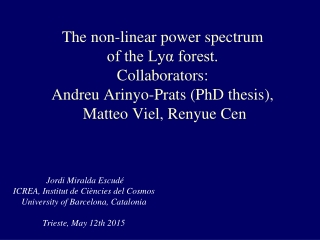DownloadDownload PresentationJohn Wheeler

John Wheeler

Télécharger la présentationJohn Wheeler

- - - - - - - - - - - - - - - - - - - - - - - - - - - E N D - - - - - - - - - - - - - - - - - - - - - - - - - - -
Presentation Transcript

1. The non-linear power spectrumof the Lyα forest.Collaborators: Andreu Arinyo-Prats (PhD thesis), Matteo Viel, Renyue Cen Jordi Miralda Escudé ICREA, Institut de Ciències del CosmosUniversity of Barcelona, CataloniaTrieste, May 12th 2015

2. John Wheeler “The real reason universities have students is to educate the professors” 1911-2008

3. Patrick McDonald “The Lyα forest ought to be like the CMB: a theory predicting the power spectrum accurately that can be compared with observations, with error bars that are believable.” PhD student at Upenn and OSU 1997-2001

4. Lyα absorption spectra in photoionized medium:fluctuating Gunn-Peterson optical depth Without thermal broadening:

5. Bias factors of the Lyα forest • In every quasar spectrum pixel: • On small scales, the variations in δF are large and strongly affected by non-linear evolution and complex physical processes. But the 3-D average over a large scale should behave linearly. • Galaxy number density fluctuation in redshift space: • Same for Lyα forest, except that a peculiar velocity gradient bias factor different from 1 is induced by the non-linear relation :

6. Whatweexpect: linear theory • For a single Fourier mode, peculiar velocity gradient: • Galaxies: • Lyα forest: • Power spectrum in redshift space: • In one dimension: Kaiser 1987; Hamilton 1998; Croft et al. 1998, 1999, McDonald et al. 2000; McDonald 2003

7. Physical bias factors • The transmission bias factors we have defined tell us how transmission varies with δ and η, but F is not zero when there is no gas. The physical bias factors are related to the variations in the absorption effective optical depth. • These bias factors are interpretable as we are used to and reflect the biased distribution of the underlying gas structures in the IGM: a value of 1 means that Lyα absorption varies with the same amplitude as mass density fluctuation.

8. Simulations in Arinyo-Prats et al. 2015: Gadget-II, CDMΛ.Ωm=0.3, σ8=0.88, ns=1 (fiducial model) • At a given redshift output, choose one axis as the line of sight and compute simulated Lyα spectra of transmission fraction F along all simulation rows. • Compute 3-D FFT of the F field. • Compute power spectrum PF(k,μk), divide by linear power PL(k) • Fit formula for the non-linear power spectrum (McDonald 2003): Ansatz from perturbation theory:

9. PF/PL : 120 Mpc/h box, 7683particles

10. Non-linear correction

11. The μ-dependence of log(D) is very close to a power-law (why?) PF/PL is nearly flat at a value of k where the non-linear anisotropy just cancels the linear one (all curves of different μ cross each other)

12. Linear biasfactors • Density bias factor is nearly flat at ~ 60%. • Velocity gradient bias is close to 1 at z ≈ 2.3 , decreases with z. • β ≈ 1.4 at z ≈ 2.3, decreases with redshift. • There is some dependence on the fitting function, which can only be resolved with more simulations to reduce uncertainties.

13. Many tests are done: box size…

14. Many tests are done: Eulerian vs. Gadget simulation…

15. Dependence on power spectrum amplitude, σ8

16. Bias factors for different σ8 • Transmission power varies weakly with mass power, so density bias factor is nearly inversely proportional to σ8. • β increases with σ8.

17. Redshiftevolution • The redshift evolution is a combination of the effect of changing <F> and the variation of the power spectrum amplitude with redshift. • These two effects fortuitously cancel for the density bias factor, resulting in a constant bτδ.arly inversely proportional to σ8. • β decreases with z owing to the decrease of bτη.

18. First year results: redshift distortions (Slosar et al. 2011) Clearly detected anisotropy, consistent with Kaiser’s linear formula. • Fitting model: • Also, from quasar-Lyα cross-correlation, β ≈ 1.2. • But continuum fitting distortion effects prevented more reliable fits.

19. Blomqvist et al. 2015: DR11 data withanimprovedmethodtodealwith continuum fittingdistortion. These linear bias factors agree with our results for a model consistent with Planck, although with substantial errors in the theory prediction at the level of 10-20% that can only be improved from the analysis of more simulations on large boxes.

20. Conclusions • We can use numerical hydrodynamic simulations of the Lyα forest to predict the non-linear form of the transmission power spectrum and the values of the linear bias factors. • These can be measured from observations and compared to the theoretical predictions. • At the moment we have only made a preliminary comparison of the linear bias factors, and they are consistent but still with large errors. • We should be able to greatly improve the reliability of the theoretical predictions for the Lyα forest power spectrum, and measure it in detail from BOSS, eBOSS and upcoming surveys (WEAVE, 4-MOST, DESI), as well as the sample of luminous quasar pairs observed at high S/N for very small scales.